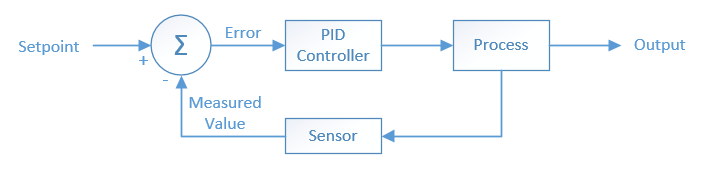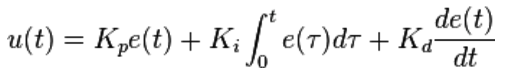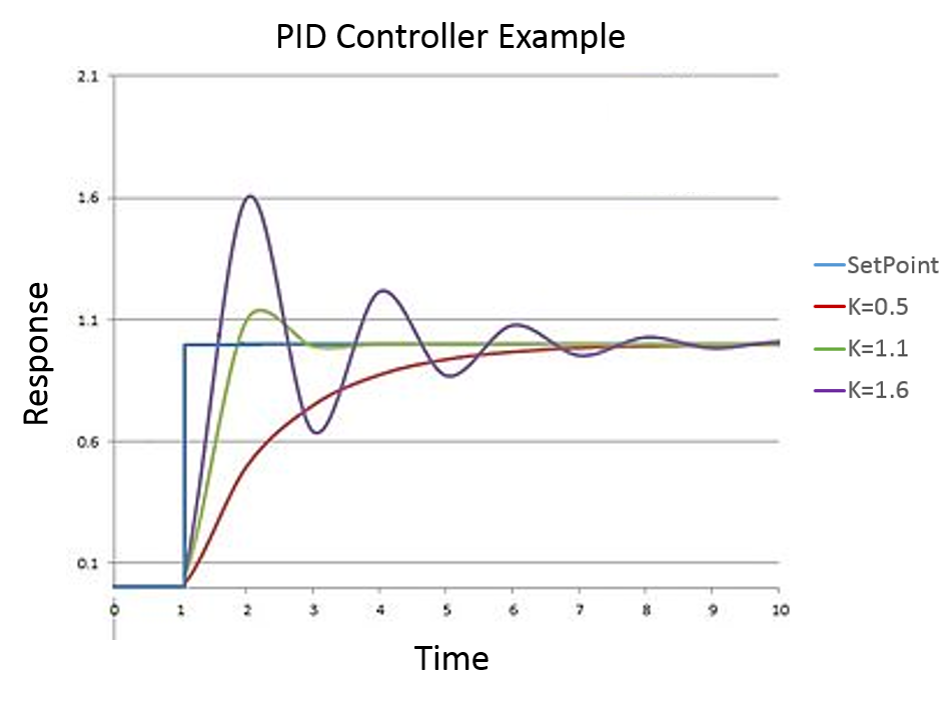# Example Tutorial: PID Controller

This project shows an example of a tutorial that would be accepted as extra credit for the diotu 2018 course

Created: March 27th, 2018

0

## What is a PID Controller?

A PID (Proportional, Integral, Derivative) controller is a feedback system, stemming from control theory, that attempts to control a system or process. The PID controller calculates the error between a desired setpoint and a measured process variable. The controller continuously attempts to minimize this error over time by adjusting an output variable and recalculating the error.

0

## PID Controller Schematic0

## PID EquationThe PID controller equation is built around 3 constants, the proportional, integral, and derivative coefficient. Each of these constants can be adjusted to achieve a desired control response.

• u(t) - Output
• e(t) - Error Value
• Kp - Proportional constant that accounts for the present error value. If the current error is large and positive, the output will be large and positive
• Ki - Integral constant that accounts for historical error values. If the output is not strong, the error will accumulate, and the controller will respond by increasing the output.
• Kd - Derivative constant that accounts for future error values. This term attempts to predict what the error will be in the future, and adjusts accordingly.
0

## PID Response GraphThe graph above shows how the response of PID controller can change based on the set coefficients. In this example, the system is subjected to a setpoint change. The controller with K = 0.5 reacts rather slowly and reaches the setpoint with no overshoot. This condition is referred to as overdamped. The controller with K = 1.6 reacts extremely fast and severely overshoots the setpoint. The controller adjusts accordingly so that the output eventually settles down at the setpoint. This condition is referred to as underdamped. Finally, the controller with K = 1.1 reaches the setpoint quickly with minimal overshoot. Each PID controller application is different than the next and the coefficients need to be adjusted appropriately to achieve the desired response.

0

## Example Applications

### Automotives:

PID controllers are useful in the automotive industry for maintaining a constant speed or separation distance during cruise control. The controller will measure the actual distance compared to the desired distance and adjust the speed in order to minimize the delta.### Robotics

PID controllers can help a robot achieve a desired movement or position. The PID controller will measure how far off the course the robot is, and adjust the steering to remain on the course.